# Simplifying Laplace’s Tidal Equations for QBO

[mathjax]I have been playing the models of ENSO and QBO off of each other. So for whatever insights I glean from one model, I have tried to apply it to the other.  My last ENSO analysis involved simplifying Laplace’s Tidal Equations and finding similarity to the Mathieu-modulation used to describe sloshing of water volumes.  However, I had left one equation in a rough state, and stated that I would get back to it.  That’s what I will do in this post, and I can flatly assert that the simplification is an eye-opener for understanding the behavior of QBO! (Which shouldn’t be surprising since Richard Lindzen tried vainly to apply Laplace’s equations to QBO, with the outcome of creating a monster of complexity, see  )

# Ridiculous Paper on Ocean Oscillation

These scientists thought that they could increase interest in their research by converting a very low frequency ocean oscillation into a human audible tone.  They then called it a “Rossby Whistle”.  As they write “this resonant mode is dynamically equivalent to the operation of a whistle.”

, , , , and (2016), A Rossby Whistle: A resonant basin mode observed in the Caribbean Sea, Geophys. Res. Lett., 43,  doi:10.1002/2016GL069573.

This is really silly because converting it to a human audible tone is meaningless, as the original frequency has a period of months, which is absurdly below the human audible detection limit of 10 to 20 Hz at the low-frequency end of the audio spectrum. And so it is only intended to illicit a response in people that might find it spooky sounding.

Here is my take on comparing a tone with harmonics of the fundamental frequency to that of a converted Quasi-Biennial Oscillation (QBO) waveform.

This is the spectrum of the harmonic tone; note the octave doubling in the peaks starting with the harmonic at 270 Hz.This is what the QBO looks like. These are not harmonics but are aliased frequencies of the original tidal frequency.This is a scope trace of the harmonic tone. The envelope is the fundamental and the harmonics provide the finer structure, forming a regular pattern.And this is a scope trace of the equivalent QBO tone, showing the more erratic nature of combining the aliased frequencies.And this is what they sound like in comparison. The first tone is the harmonic tone, and then separated by a pause is the QBO tone.  The first sounds pleasing and the second converted QBO waveform sounds out-of-tune because the frequencies aren’t harmonics.

As John Gielgud once said, “I’ll alert the media”. Sigh. In our current media circus,reporters will lap up the inconsequential aspect of the strange sound and ignore the fact that Richard Lindzen couldn’t figure out that the QBO comes about from seasonal aliasing of the lunar nodal cycle.  Even though he knew that a lunar tidal mechanism could form a valid premise for atmospheric oscillation.Example of the second-derivative of the QBO, which exaggerates the aliased frequencies.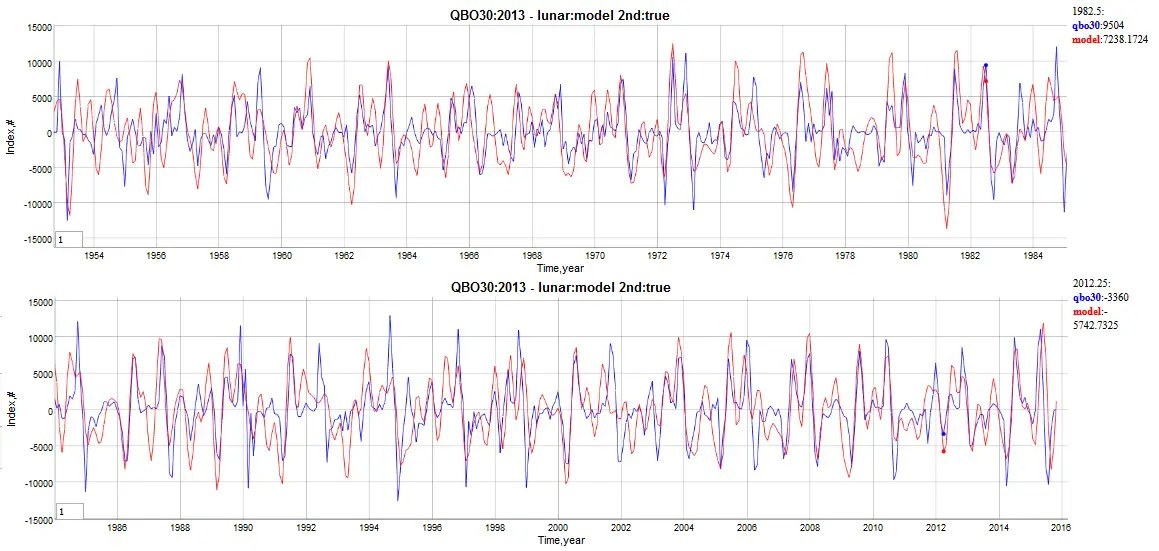Still can’t figure out why Lindzen never saw this.

# Recent research say QBO frequency is emergent property

Gavin Schmidt of NASA tweeted a cite to a recent paper on QBO by a group of 12 scientists

Geller, M. A., Zhou, T., Shindell, D., Ruedy, R., Aleinov, I., Nazarenko, L., Tausnev, N.L., Kelley, M., Sun, S., Cheng, Y., Field, R.D. and Faluvegi, G. (2016), Modeling the QBO – Improvements Resulting from Higher Model Vertical Resolution. J. Adv. Model. Earth Syst.. doi:10.1002/2016MS000699

They claim that the fundamental frequency of QBO relates inversely proportional to the pressure:

“The mean zonal winds from the ERA-Interim reanalysis are shown between 4.5 and 4.5 N between heights of 100 and 1 hPa for the 20 years 1991-2010. Note that ERA-Interim shows that approximately 8 1/2 QBO cycles occurred during this period, implying a QBO period averaging about 28 months. The model results in figure 1b show that no coherent QBO resembling observations exists for the gravity wave momentum flux forcing of 1.5 mPa, which is consistent with the steady jets at low wave forcing demonstrated by Yoden and Holton (1988). It also shows that the QBO-like oscillation for a gravity wave momentum flux forcing of 2.0 mPa has a period of about 8 years, while a forcing of 2.5 mPa gives a period of about 37 months, and a forcing of 3.0 mPa gives a period of about 25 months, and a forcing of 3.5 mPa gives a period of about 21 months. In fact, we find that the best fit to observed QBO periods is for a gravity wave momentum flux forcing of 2.9 mPa, as will be shown in the next section. “

I disagree with this interpretation. I know that this might sound like I am using the argument of personal astonishment, but I can’t see how a physical model could operate this way. If anything, pressure may modify the amplitude of the oscillations, i.e. the peak speed, but asserting that the pressure sets the oscillation period is likely the result of a model that no one understands. If it was indeed caused by the pressure, they should be able to come up with a simplified formula, like deriving the resonant frequency of a Rijke tube, instead of suggesting that it is an emergent property of a complicated model. Certainly, pitch can change as a result of say overblowing a woodwind instrument, but even there, the shifts are more than likely harmonics or overtones of the original fundamental frequency, and not a continuous change in frequency.RHEED oscillations more here

And I realize that the QBO is obviously not an acoustic oscillation yet the physical model for any oscillation does need some sort of mathematically intuitive explanation (see figure to the right), which is greatly lacking in this paper.  To say it emerges from the model is an extremely weak argument.

The 28 month period is ubiquitous in a number of geophysical oscillations. It shows up in (1) the seasonally aliased Draconic lunar cycle, (2) the strongest factor in the model of ENSO, (3) exactly half that period in the Chandler Wobble, (4) its defined as the mean annual flood recurrence interval by the USGS (first reported in 1960), and (5) the fundamental QBO period.

Perhaps no one wants to admit that seeing this number show up in all these measures is most likely a forced response from the nodal lunar cycle factor, as opposed to a natural resonance. The reality is that a forced response is not as “sexy” as finding a natural frequency eigenvalue.

But of course there is no way to verify either way, since a controlled experiment is not available to test a model against.  There is only one earth and it can’t be duplicated in the lab. All we have to go on is the empirical data and any model that we can dream up.

A bunch of meteorologists are freaking out that the current QBO data is not meeting up to expectations

I don’t understand the rarity of this since the higher altitude QBO aligns more with semiannual seasonal cycles, while the lower altitude QBO aligns more with the nodal lunar cycles. These will constructively add according to a beat frequency and so the alignment will occur occasionally. This summer solstice there is a full moon and that only happens once every 19 years.  I can see this with my QBO model as well, so what is the big deal?

# Model of ENSO

[mathjax](update here)

Putting the finishing touches on the ENSO model, I decided to derive the Mathieu modulation of the wave equation. It’s actually a rather obvious simplification of Laplace’s Tidal Equations or the Shallow-water Wave Equation applied along the equator. That last assumption is important as the Coriolis forces are known to cancel at the equator (added: perhaps more precisely Isaac Held explains that it vanishes at the equator — it is both zero and a reversal) and so we can use a small angle approximation to reduce the set of equations to a Mathieu or Hill formulation.

To make the job even easier, the three equations that comprise the the Laplace formulation can be formulated initially in terms of the Laplace tidal operator. Per the recent reference , the authors separate the temporal component from the standing-wave spatial component(s) and express the operator concisely via implicit time and wavenumber (see Figure 1).

Although the paper proceeds to detail the computational approach to solving the tidal equation, we will only piggy-back off the formulation and arrive at a gross simplification.

The first step is to use the small angle approximation to $$phi$$$mu = sin phi congphi$

If $$sigma gg mu$$ while $$sigma$$ not a function of $$mu$$, then the operator can be reduced to:$F(Theta) = frac{1}{sigma^2} left( frac{d^2Theta}{dmu^2} - left[frac{s}{sigma} + s^2right] Theta right)$

The next crucial step is to extract the implicit temporal change from $$mu$$ via the chain rule.  Even though $$mu$$ is small, changes in $$mu$$ have to be retained to first-order or else the solution would be static along the equator.$frac{d^2Theta}{dmu^2} = frac{d^2Theta}{dt^2} frac{d^2t}{dmu^2}$

added 6-17-2016: In spirit, the above is close, but not a correct expansion.
The actual chain-rule expansion is a bit more complicated and features first-derivative cross-terms.
Will get to that later, not a big deal.


The second term has the dimensions of a reciprocal latitudinal acceleration. If we assume that $$mu = mu_0 sin(omega t)$$, then$F(Theta) = - frac{1}{sigma^2} left( frac{d^2Theta}{d t^2} frac{1}{mu_0 omega^2 sin(omega t)} + left[frac{s}{sigma} + s^2right] Theta right)$

With that simplification, we reduce the formulation to a temporal second-order differential equation.$frac{1}{sigma^2} left( frac{d^2Theta}{d t^2} frac{1}{mu_0 omega^2 sin(omega t)} + left[frac{s}{sigma} + s^2right] Theta right) + gamma Theta = f(t)$

where f(t) is a forcing added to the right-hand side (RHS).

Moving the $$sin(omega t)$$ from the denominator, we recover the Mathieu-like wave equation formulation:$frac{d^2Theta}{dt^2} + left[frac{s}{sigma} + s^2 + sigma^2 gamma right] Theta mu_0 omega^2 sin(omega t) = sigma^2 mu_0 omega^2 sin(omega t) f(t)$

For now, the primary objective is to verify the sinusoidal behavior in terms of correlating periodicities. Previously, we determined that the f(t) term was composed of seasonally aliased lunar periods, such as the Draconic 27.2 day cycle. (Recently I also found this term buried in the LOD data.)

And the Mathieu modulation likely includes an annual contribution, and, importantly, a strict biennial cycle $$omega=pi$$, which is both observed and implied based on other climatic measures.

The seasonally aliased 27.2 day cycle generates harmonics in ENSO at the same locations as for QBO
1/T = 1/P + n, where P is the tidal period in years, and n is … -3, -2, -1, 0

so for Draconic, 1/T = 365.242/27.2 = 13.43. Stripping off the integer, the value of 0.43 is apparent (and 0.43-1=-0.57) as the strongest low-frequency factor in the power spectrum of Figure 2. Similarly for the fortnightly Draconic (13.6 day), 1/T = 365.242/13.6 = 27.85, and stripping off the integer, one gets 0.85 (and 0.85-1=-0.15). This also corresponds to the Chandler wobble. And with the multiplicative biennial modulation factor adding a +/- 0.5 to each aliased value, the other candidates are 0.85-0.5 = 0.35, 0.43+0.5 = 0.93 (and -0.57+0.5=-0.07, -0.15-0.5=-0.65). All these values are observed as among the strongest low-frequency components in the power spectrum below.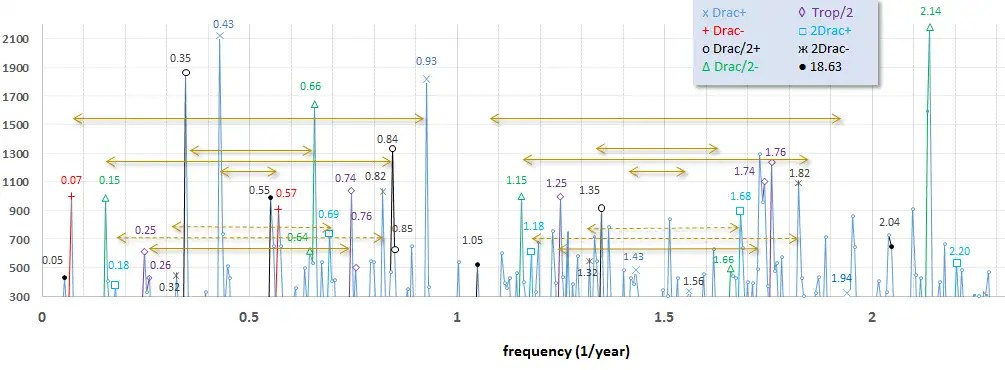Fig. 2:  The strongest components correspond to the seasonally aliased Draconic period. The arrows separate the biennial symmetric terms.

The model fit to the ENSO spectrum is shown in Figure 3. This includes more than the Draconic terms, including potential Tropical fortnight (27.32/2 day) and Anomalistic (27.55 day) terms. Apart from the 1/f filtering envelope, the symmetry about the f=0.5/yr is evident. Note that the power spectrum calculated is essentially the LHS response (data) against the RHS forcing (model). The second derivatives and modulation are included in the LHS, so it is not completely “raw” data.Fig. 3:  ENSO power spectrum comparison to model

And in temporal space, the multiple regression fit is shown in Figure 4. Interesting that the correlation coefficient is higher outside the training interval than within the training interval!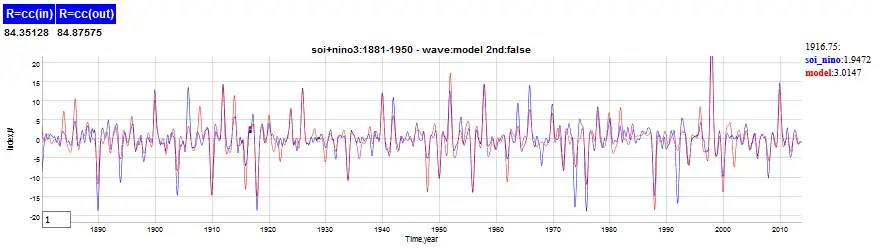Fig. 4: Fit using the training interval from 1881 to 1950.

This is a very elegant theory in terms of deriving from first-principles; the fact that it is a simplification direct from Pierre Laplace’s original tidal formulation means that we have bypassed sophisticated GCM approaches to potentially solving ENSO .  The only caveats to mention are the phase inversion from 1981 to 1996, and the imprecision of the  Draconic term, where 27.199 days fits better than 27.212 days.  The former aligns with a 7 year interval as there are precisely 94 cycles of 27.199 day periods in 7 years.  As ENSO is known to be seasonally aligned, this makes qualitative sense. However, the phase error from that cycle to the actual Draconic cycle will build up over time, and it will eventually go completely out of phase after 70+ years.  This actually may be related to the 1981 phase inversion, as that would be a means of re-aligning the phase after a number of years (Another phase inversion may occur prior to 1880, see comment).

The white paper is located on arXiv.

I have to get to work so will leave this post subject to fixing any Latex markup typos later. (Note: fixed some sign-carryover errors due to the 2nd-deriv, same day as posting)

## References

 Wang, Houjun, John P. Boyd, and Rashid A. Akmaev. “On computation of Hough functions.” Geoscientific Model Development 9.4 (2016): 1477. PDF

# QBO Aliasing Graph

Before getting to the needless complexity discussion hinted at in the last post, here is a simple graphical analysis of finding aliased frequencies. Based on this analysis one can determine aliased signals by looking at the frequency power spectrum. The rules for aliasing in frequency space involve peeling back from the assumed frequency until the aliased harmonics appear in the spectrum.

1/T = 1/P + n, where P is the tidal period in years, and n is … -3, -2, -1, 0

So applying the Draconic period aliasing to QBO, the harmonics are clearly seen in the following Figure 1.  The strongest factor is the approximately 2.33 year period shown on the left, but since this is a second derivative of the QBO time series, higher frequency aliased harmonics are also observed to the right.  Note that the factors are approximately 0.43, 1.43, and 2.43, which are separated by Δn=1. And note that 1/0.43 ~ 2.33 years, which is the approximate observed QBO frequency..Fig. 1:  Spectrum of the second derivative of QBO, showing seasonally aliased factors

Other potential periods are shown in the figure, including the unaliased annual and semi-annual signals separated by the violet colored arrow. The green arrow separates the harmonics of the 13.633 day lunar tide periodicity. Clustered around the strong Draconic period are also satellite frequencies. At least one of these satellites along with the strong aliased Draconic also appear in the LOD spectrum. That provides the physical basis for QBO (and also ENSO) forcing.

Putting these factors together via multiple regression results in marvelous fits of the various QBO time series, as seen in Figure 2 below.Fig. 2:  Multiple regression fit of the 30 hPa QBO time series.

And to highlight the second derivative composition and how the higher frequency aliased harmonics contribute to the fine detail, see Figure 3.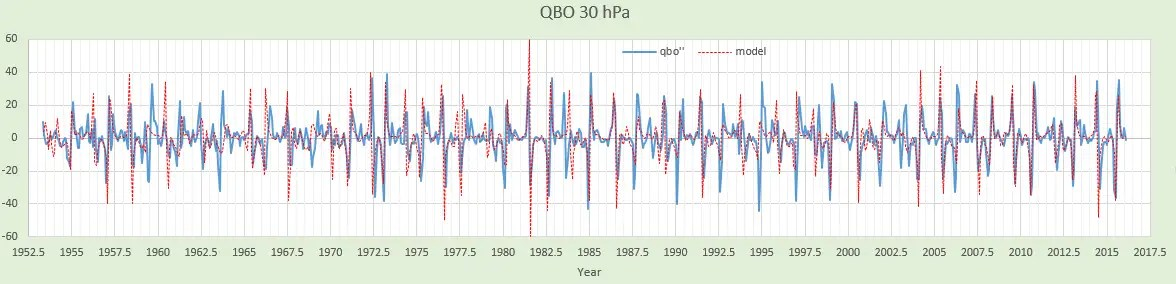Fig. 3 : Second derivative of QBO highlights the higher frequency aliased harmonic detail.

Only in a few places do the aliased harmonics not appear well aligned. Those years, such as the interval 1983-1984 may be telling us that other climate events may be playing a role in temporarily impacting the QBO dynamics.

# Seasonal Aliasing of Long-Period Tides Found in Length-Of-Day Data

From a careful analysis of Length-of-Day (LOD) measurements, we can glean lots of information about phenomenon such as tides, earthquakes, core/mantle motion, and ENSO .  In Figure 1 below, one can see how this is manifested at different time scales.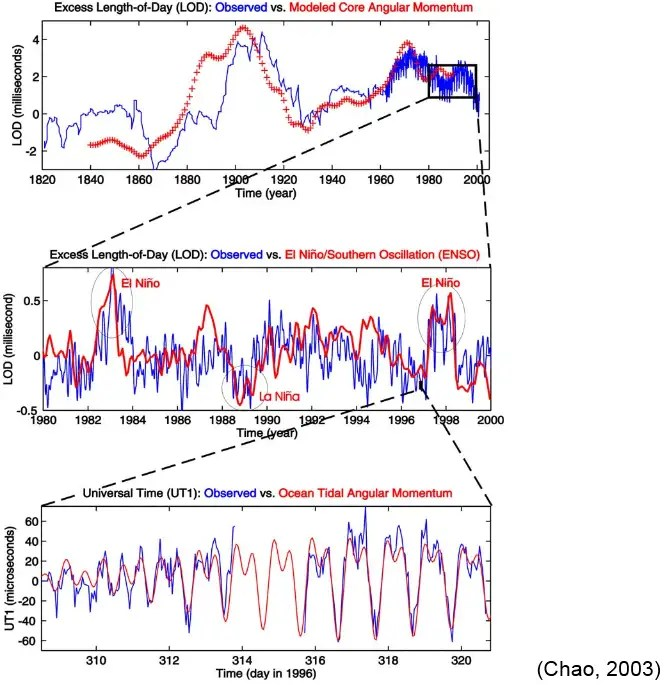Fig. 1: Differing temporal scales of LOD analysis.  Short period tides have scale of diurnal and semidiurnal.

I revisited the LOD data to see if I could detect the angular momentum changes that appear to force the QBO and ENSO oscillations, similar to what Wang et al do in .  In each of these natural behaviors, there is a clear indication that the 2.369 year seasonally-aliased long period draconic tide is a primary forcing mechanism.

There are two sets of data to look at. The original set from Gross at JPL called LUNAR97  is not online in a machine-readable format, but it is included in the paper. So it was a simple matter to OCR a GIF of the paper’s table. Another more recent set is available from IERS here.Fig. 2: Compariscon between the two LOD data sets.  The Gross set has less filtering

The issue with the more recent set is that the site claims that they do a “5-point quadratic convolute”, at least up to 1955.  I don’t want any filtering, so chose the Gross LUNAR97 set. You can see the difference between the two data sets in Figure 2 above.

To draw out the fine features even more, I performed a straightforward second-derivative on the LUNAR97 time-series (same approach as used on the QBO see result). This was then fed into a symbolic regression machine learning tool to determine the principle components. The results are shown in Figure 3 below.Fig. 3:  Result of machine learning on 2nd-derivative of LOD data.

After about an hour of machine learning, the solution included a strong frequency at 22.481 rads/year.  In Figure 3, that solution was one of the simplest along the Pareto front, shown by the red dot. This frequency is aliased to the slower frequency of 2.3694 rads/year, which is correspondingly seasonally aliased to an approximate lunar month of 27.2121 days. Incredibly, but perhaps not surprisingly, this compares to the known draconic or nodal lunar month length of 27.21222 days.   The other somewhat weaker term is very close to aliasing the anomalistic lunar month of 27.55 days, in the fortnight mode of 13.777 days.

That was the first run I attempted and subsequent runs are picking out some other potential long-period lunar periods (and also the 6.45 year Chandler wobble), but this is really enough to demonstrate a salient result — that the same draconic period that the QBO model uses and that the ENSO model uses as the primary angular momentum forcing term also appears in the most direct and practical measurement of angular momentum known in Earth geophysics.   And I do not think this is a instance of artificial sampling aliasing, since each of the LOD measurements was averaged over at least a month.  It is likely a real seasonal aliasing behavior and serves to further substantiate the theorized mechanisms forcing the QBO and ENSO behavior.   Read the short paper that I placed into arXiv to see how the other parts fall into place.

And a continuing lesson to be learned is to not filter data unless you can be sure that what you are filtering out is nuisance noise. In this case, it appears the filtering performed by IERS was too aggressive and removed a very important signal in the time series! Contrary to what the statistician Tamino has been preaching, these time-series do not have a lot of statistical content. I donated money to his lessons on time-series analysis, but largely disagree on the applicability of his approaches to earth data such as we see in QBO, ENSO, Chandler wobble, and now LOD. If you followed his instructions, you might not find any of the periodic cycles buried in the data, and you might dismiss it all as red noise. Yet, there is likely nothing statistical or complex about these behaviors, as they are largely driven by known deterministic forces. Stay tuned, as the next post will be titled “Needless Complexity”.

## References

 B. Fong Chao, “Excitation of Earth’s polar motion by atmospheric angular momentum variations, 1980–1990,” Geophysical research letters, vol. 20, no. 3, pp. 253–256, 1993.

 Shen, Wen-Jun Wang Wen-Bin, and Han-Wei Zhang. “Verifications for Multiple Solutions of Triaxial Earth Rotation.”  PDF

Note: the authors in  also find a 14-year signal in the LUNAR97 data (see figure below). This is likely a result of a wavelet filter that isolated periods between 8 and 16 years as they described the windowing procedure.  This 14 year period is critical in the ENSO model.From Wang et al, Figure 6 “Spectrum of wavelet for data series LUNAR97 with evident peak at period 14 yr “.   The reciprocal of 0.0715 cycles/year is 14 years.

 Gross, Richard S. “A combined length-of-day series spanning 1832–1997: LUNAR97.” Physics of the Earth and Planetary Interiors 123.1 (2001): 65-76.  PDF